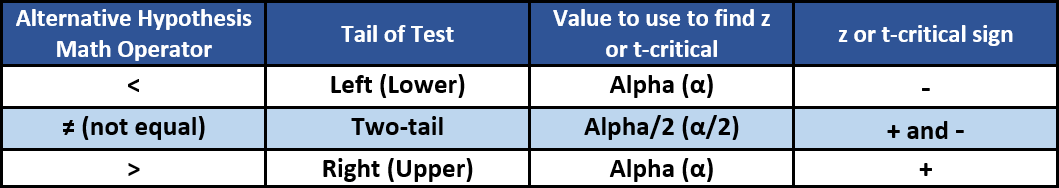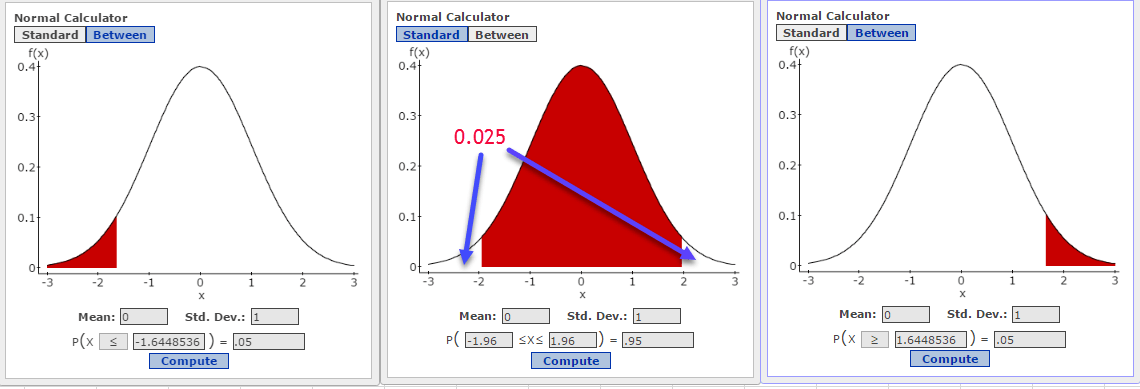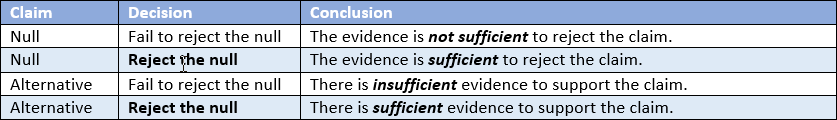# Setting up Hypothesis Tests

The Null and Alternative
The most common problem I noticed on this assignment was caused by failing to properly identify the appropriate null and alternative hypotheses. In part, this is due to the Evans text’s somewhat confusing explanation of how to do this – the “burden of proof” approach. There is a much simpler approach that always works.

First, make sure you closely read the problem statement looking for key words and phrases. This table may help:The null hypothesis always is “no effect” or “no difference.” The null says that nothing new happened or the groups have the same mean – the status quo. The null states that a population parameter is equal to some value. It always is written with an equality statement, i.e. =, ≥, ≤. We always assume the null to be true.

The alternative states that the population parameter is different than the value in the null. It often is what is believed to be true or what we hope to prove. The alternative is the complement of the null and always contains an inequality statement, i.e. ≠, <, >. Initially, the alternative is assumed to be false, but it might be accepted if sufficient evidence is obtained.

Next, decide which of the hypotheses represents the claim. The claim can be either the null or the alternative. This is where the “burden of proof” framework is suggested. The rational is based on the underlying principle of hypothesis tests that the null is always assumed to be true but cannot be proven to be true. Based upon the evidence obtained, we can statistically “reject” the null if we have sufficient evidence supporting the alternative. But, because of the possibility of having sampling errors (random occurrences) in our data, we can never prove the null is true, only that we failed to get sufficient evidence to support the alternative; thus we “fail to reject” the null.

On the other hand, we can hope to gather sufficient evidence to support the alternative hypothesis. If we do, we can then “reject the null.” If the null is rejected, the alternative is accepted as being true.

Under the burden of proof conceptualization, if we want to prove the claim, we have to make it the alternative. However, this approach becomes problematic if the claim in the problem statement contains an equality, as this means the claim must be the null. Forcing the claim containing an equality to be the alternative forces us to change the actual wording of the problem statement. And that can lead to confusion and mistakes in setting up and running the actual hypothesis test.

My suggested approach is to always set the null and alternative first, and then decide which one is the claim. Although we cannot prove the claim to be true if it is the null, we can get evidence which will allow us to “fail to reject” it. In a practical (though not technical) sense, this is effectively the same thing.

Direction of the testYour test can be left/lower-tailed, right/upper-tailed, or two-tailed. The direction is determined by the alternative hypothesis. If the mathematical statement of Ha contains <, the symbol is pointing to the left and you have a left-tailed test. Same thought process for Ha containing >. If Ha contains ≠, you have a two-tailed test.

It always helps to draw a sketch of the problem showing the critical areas, also called rejection regions, of the distribution. The following three graphs show rejection areas for a left-tail, two-tail, and right-tail tests for an alpha of 0.05 (95% confidence level). I created them using StatCrunch’s distribution calculator for the normal distribution – a z-test. You would have something similar for a t-test or a Chi-square test. For a left-tail test, the rejection region is always on the negative (lower) side of the mean. This is the red area in the first graph and represents a probability of 0.05. You can think of it as all of alpha is put in the left tail. As shown in the first graph, the critical value of z for this test is -1.645. The far right graph is of a right-tail test and, again, all of alpha is put in that right tail. For a right-tail test, the critical value of z is always positive, here equal to 1.645. The middle graph is a two-tailed test which means half of alpha, or 0.025, is placed in each tail. A two-tail test has two rejection regions, this time the white areas in the two tails, and we have two critical values of z, -1.96 and +1.96.Z-test or t-test or …?
First question: am I dealing with means or proportion? Proportion problems are always a z-test IF np and n(1-p) are both >10. If not, you have to run either a binomial or hypergeometric test.
Next question: Do I know the population standard deviation, sigma (σ) ? Remember the sample standard deviation is not the population standard deviation σ. If you know σ, run a Z-test. Otherwise, run the t-test.

State the Conclusion

For this course and even later in your careers, if you conduct a hypothesis test, you must make a clear statement of the conclusion you reach. It is not sufficient to just say “Reject the Null” or “Fail to reject the null.” Just as you might have had a difficult time reading the problem and setting up the test, your readers might not be sure what your test results really were.
Be sure to include these things:
1. Significance level, α. Remember the test is set to recognize an acceptable level of uncertainty – the significance level, e.g. 5% of the time we will get as extreme or more extreme results purely by chance.
2. State the actual p-value and compare it to α. Alternatively, calculate the critical value of z or t, identify the rejection area, and decide if the standardized test statistic falls in the rejection area.
3. Evidence. Is there sufficient evidence to reject the null/support the alternative.
4. Claim. This is pretty straightforward.5. Do not include extraneous statements such as “The actual mean was 4100 which is obviously different than the hypothesized 4500.” The measured mean only makes this sense if you sample the entire population. If you do, you don’t need a hypothesis test.

This site uses Akismet to reduce spam. Learn how your comment data is processed.